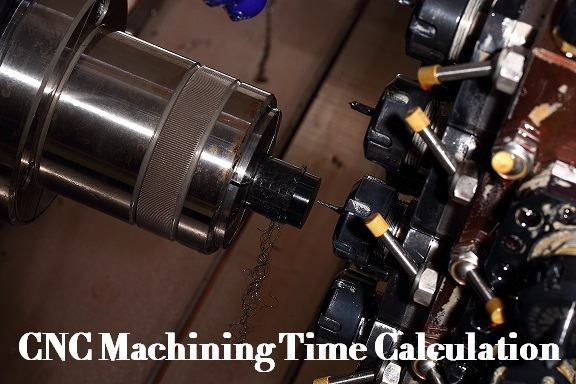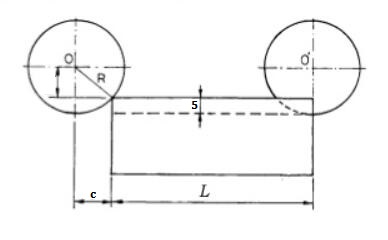> > How to Calculate CNC Machining Time – CNC Machining Cycle Time Calculation | CNCLATHING
> > How to Calculate CNC Machining Time – CNC Machining Cycle Time Calculation | CNCLATHING

# How to Calculate CNC Machining Time – CNC Machining Cycle Time Calculation | CNCLATHING

2020.12.16

In the actual CNC machining process, it is often required to determine how long one or several operations will take to complete, which is related to the machining cost and lead time of the end product. The calculation formula of CNC machining cycle time is easy, but the situation in the real calculation is much more complex, especially in different operations such as turning and milling. So in this article, follow us to figure out how to calculate CNC machining time for the milling process with a detailed example, then understand the turning/milling machining time formula better.## How to Calculate CNC Machining Time - CNC Machining Cycle Time Calculation Formula for Turning, Milling and More

From the most basic rule, the CNC machining time is similar to other times, that is the distance or length divided by the speed or rate. Combines the characteristics of machining processes, the general formula for CNC machining time calculation of turning, milling, facing and more operations is as below. The machining time here refers to cutting time.

T=L/f*N

N=1000*V/π*D

T=Machining Time

L=Length of cut in mm

N= rpm (revolution of the job per minute)

V=cutting speed in mpm (meters per minute)

D=diameter of the rod in mm

f= feed in mm/rev (millimeters per revolution)

## CNC Milling Machining Time Calculation

CNC milling is a machining process can make slots or ribs of various sections, flat surface in vertical, horizontal and inclined planes, surfaces of revolution, helical grooves and more. For milling operations, the feed rate may be in per tooth, which means that you need to know the number of cutting edges, teeth, or flutes on the tool. Here let’s see an example of how to calculate the milling machining time.

Milling Machining Time Calculation Example:

A slot of 25*25mm is milled in a part of 300mm length using a side and face milling cutter of diameter 100, width 25mm and 20 teeth, the depth of cut is 5mm, feed per tooth is 0.1mm and cutting speed is 35m/min, tool approach and over travel distance of 5mm each, how long it requires?

In this question, we need to calculation the machine time of the milling process, the original formula is T=L/f*N, how to get each value in the formula?

1. L (Length)

Firstly, the depth of cut is 5mm, which means the tool only can cut 5mm in one time, so to cut 25mm, the tool needs to do it 5 times, then the number of passes is 5. The formula will become T=L*No. Of passes/f*N

Secondly, the length in this question is obviously not 300, it has to cover the tool approach and over travel as well, in addition, since the depth of cut is not equal to the radius of the milling cutter and it is smaller than the radius, so the point of contact will below the horizontal level of the center, if you want to start machining this material, you can’t directly start from the bottom part because you will bring the cutter firstly touch the top point, the whole depth will not be engaged by the cutter initially, what you need to do is push the cutter into the workpiece up to a certain distance, after that, it can fully engage with the depth then start machining, this distance is the compulsory distance (c in the following picture), which needs to be added to the length, compulsory distance²=50²-(50-5)², so the compulsory distance=21.79, so the L=length of the job+tool approach+tool over travel+compulsory distance=300+5+5+21.79=331.79mm.2. f (feed) and N (rpm)

The total time is a total distance to be traveled divided by the speed at which is it traveling multiplied by the number of times it has to travel. So the next thing we need to figure out is the speed in mm/min, which is feed multiply RPM. So the f=feed per revolution=feed per tooth * number of teeth = 0.1 * 20 = 2mm, the RPM is N=1000* cutting speed/π*D=1000*35/3.14 * 100=111.46

3. T (CNC milling machining time)

Finally, the T=L*No. Of passes/f*N=331.79*5/2*111.46=1658.95/222.92=7.44 minute.

## CNC Turning Machining Time Calculation

Turning is a machining process in which producing turned parts by a single point tool on lathe machines. The cutting tool is fed either linearly in the direction parallel or perpendicular to the axis of rotation of the workpiece, or along a specified path to manufacture complex rotational shapes. The primary motion of cutting in turning is the rotation of the workpiece, and the secondary motion of cutting is the feed motion. When calculating or estimating the CNC machining cycle time for the turning process, the formula is also based on T=L/feed*N, the L or length=(tool approach+job length+tool over travels)*No. Of passes, the N refers to average RPM, which is equal to 1000*cutting speed/π*average diameter.